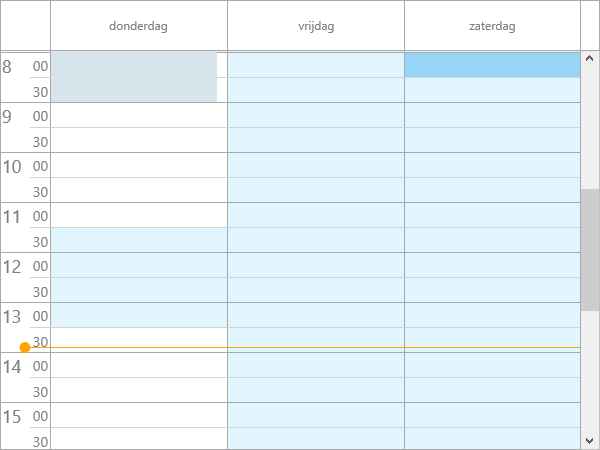# FNC Planner

Hello,
Is it possible to have differnt TimeLine.ActiveStart & TimeLine.ActiveEnd for differnt Resouce in a Planner?

I ahve not been able to find any reference to this in the documentation.

Regards
David

Yes, you can do this in the OnIsDateTimeInActive event.

Hello Pieter,

Thank you for your feedback - I will give that a go.

Welcome, if anything comes up, please let me know.

Hello,

Unfortunalrty I am not having much luck in getting this to work.
Would you by any chance have any code examples?

Thank you

Sure, here is a code snippet, based on a default planner.

``````uses
DateUtils;

procedure TForm1.TMSFNCPlanner1IsDateTimeInActive(Sender: TObject;
ADateTime: TDateTime; APosition: Integer; var AInActive: Boolean);
var
dt: TDateTime;
begin
dt := TMSFNCPlanner1.ModeSettings.StartTime;
case APosition of
0:
begin
AInActive := AInActive or ((CompareDateTime(ADateTime, Int(dt) + EncodeTime(11, 30, 0, 0)) in [GreaterThanValue, EqualsValue]) and
(CompareDateTime(ADateTime, Int(dt) + EncodeTime(13, 30, 0, 0)) = LessThanValue)) or (CompareDateTime(ADateTime, Int(dt) + EncodeTime(18, 30, 0, 0)) in [GreaterThanValue, EqualsValue]);
end;
1:
begin
AInActive := AInActive or ((CompareDateTime(ADateTime, Int(dt) + EncodeTime(11, 30, 0, 0)) in [GreaterThanValue, EqualsValue]) and
(CompareDateTime(ADateTime, Int(dt) + EncodeTime(13, 30, 0, 0)) = LessThanValue)) or (CompareDateTime(ADateTime, Int(dt) + EncodeTime(20, 30, 0, 0)) in [GreaterThanValue, EqualsValue]);

AInActive := AInActive and not (CompareDateTime(ADatetime, Int(dt) + EncodeTime(7, 0, 0, 0)) = EqualsValue);
AInActive := AInActive and not (CompareDateTime(ADatetime, Int(dt) + EncodeTime(7, 30, 0, 0)) = EqualsValue);
end;
2:
begin
AInActive := AInActive or ((CompareDateTime(ADateTime, Int(dt) + EncodeTime(11, 30, 0, 0)) in [GreaterThanValue, EqualsValue]) and
(CompareDateTime(ADateTime, Int(dt) + EncodeTime(13, 30, 0, 0)) = LessThanValue)) or (CompareDateTime(ADateTime, Int(dt) + EncodeTime(16, 30, 0, 0)) in [GreaterThanValue, EqualsValue]);
end;
end;
end;
``````many thanks - That has helped allot.